# 【平价数据】GAN用于半监督学习

Salimans, Tim, et al. “Improved techniques for training gans.” Advances in Neural Information Processing Systems. 2016.

# 概述

GAN的发明者Ian Goodfellow2016年在Open AI任职期间发表了这篇论文，其中提到了GAN用于半监督学习(semi supervised)的方法。称为SSGAN

## 半监督学习

wiki上的这张图很好地说明了无标记样本在半监督学习中发挥作用：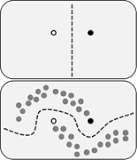## 核心理念

• 无标记样本没有类别信息，无法训练分类器；
• 引入GAN后，其中生成器(Generator)可以从随机信号生成伪样本；
• 相比之下，原有的无标记样本拥有了人造类别：真。可以和伪样本一起训练分类器。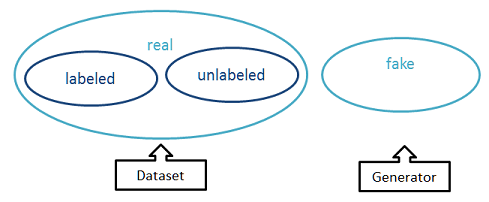# 原理

## 框架

GAN中的两个核心模块是生成器(Generator)和鉴别器(Discriminator)。这里用分类器(Classifier)代替了鉴别器。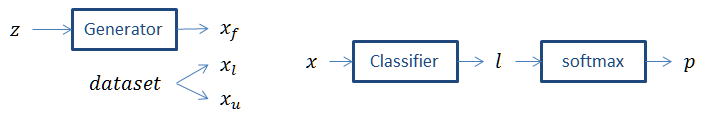p$p$的最大值位置对应为估计标签y$y$

softmax(xi)=exp(xi)jexp(xj)

## 三种误差

Llabel=E[lnp(y|x)]

Lunlabel=E[ln(1p(K+1|x))]

Lfake=E[lnp(K+1|x)]

## 推导

softmax(xic)=exp(xic)jexp(xjc)=exp(xi)/exp(c)jexp(xj)/exp(c)=softmax(xi)

Lunlabel=ln[1p(K+1|x)]=lnKj=1expljKj=1explj+explK+1=lnj=1Kexplj+ln1+j=1Kexplj

Lfake=ln[p(K+1|x)]=ln1+j=1Kexplj

Llabel=ln[p(y|x,y<K+1)]=lnexplyKj=1explj=ly+lnj=1Kexplj

LSE(x)=lnj=1expxj

softplus(x)=ln(1+expx)

Llabel=ly+LSE(l)

Lunlabel=LSE(l)+softplus(LSE(l))

Lfake=softplus(LSE(l))

## 优化目标

LD=Llabel+w2(Lunlabel+Lfake)

LG=Lfake

# 实验

## MNIST

10分类问题，图像为28*28灰度。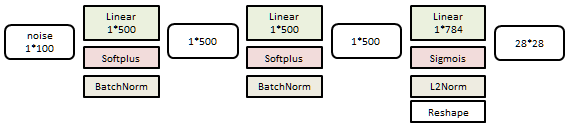## Cifar10

10分类问题，图像为32*32彩色。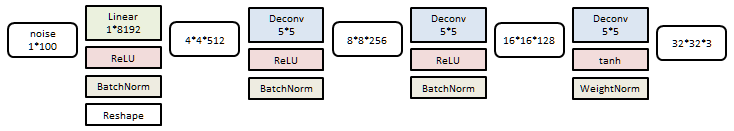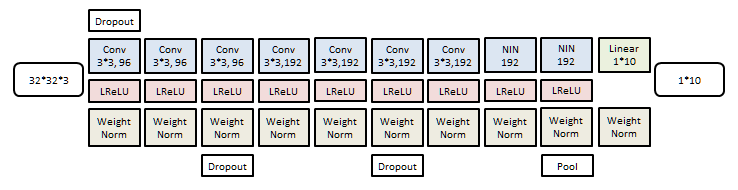## SVHN

10分类问题，图像为32*32彩色。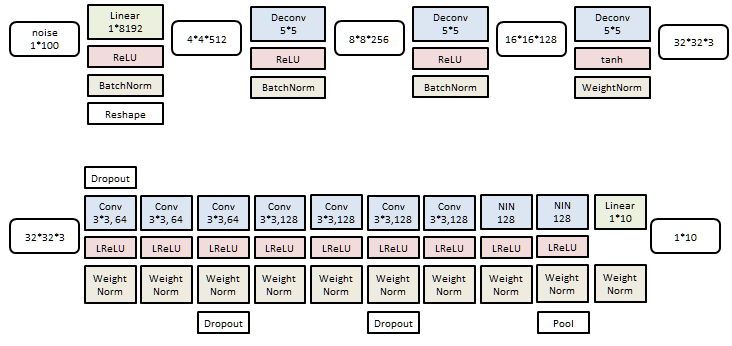1. USC的Shao-Hua Sun也给出了一个Tensorflow实现。但没有处理训练集中的无标签样本，个人认为对原文理解有偏差。

©️2019 CSDN 皮肤主题: 大白 设计师: CSDN官方博客